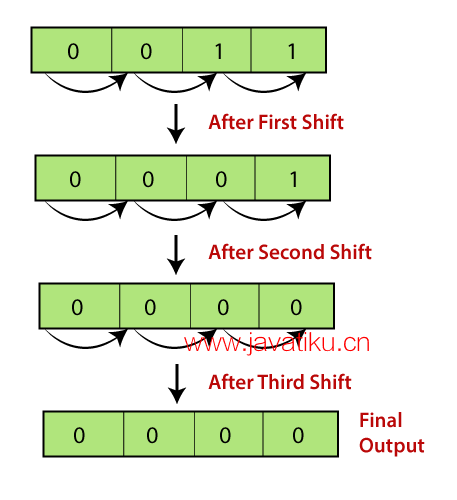# C++教程-C++的表达式C++表达式由运算符、常量和变量组成，按照语言的规则排列。它还可以包含返回值的函数调用。表达式可以由一个或多个操作数、零个或多个运算符组成，以计算一个值。每个表达式都会产生某个值，该值通过赋值运算符赋给变量。

C++表达式的示例：

``````(a+b) - c
(x/y) -z
4a2 - 5b +c
(a+b) * (x+y)``````

### 表达式可以是以下类型：

• 常量表达式
• 整数表达式
• 浮点表达式
• 指针表达式
• 关系表达式
• 逻辑表达式
• 位表达式
• 特殊赋值表达式### 常量表达式

• 在下标声明符中用于描述数组界限。
• 在switch语句中的case关键字后使用。
• 在enum中作为数值使用。
• 指定位字段宽度。
• 在预处理器#if中使用。

x = (2/3) * 4(2/3) * 4
extern int y = 6767
int z = 4343
static int a = 5656

``````#include <iostream>
using namespace std;
int main()
{
int x; // 变量声明。
x=(3/2) + 2; // 常量表达式
cout<<"x的值为："<<x; // 显示x的值。
return 0;
}``````

#### 输出

``x的值为：3``

### 整数表达式

``````(x * y) -5
x + int(9.0)
where x and y are the integers. ``````

``````#include <iostream>
using namespace std;
int main()
{
int x; // 变量声明。
int y; // 变量声明
int z; // 变量声明
cout<<"输入x和y的值";
cin>>x>>y;
z=x+y;
cout<<"\n"<<"z的值为："<<z; // 显示z的值。
return 0;
}``````

#### 输出

``````Enter the values of x and y
8
9
Value of z is :17 ``````

``````#include <iostream>
using namespace std;
int main()
{

int x; // 变量声明
int y=9; // 变量初始化
x=y+int(10.0); // 整数表达式
cout<<"x的值为："<<x; // 显示x的值。
return 0;
}``````

#### 输出

``Value of x : 19``

### 浮点表达式

``````x+y
(x/10) + y
34.5
x+float(10)``````

``````#include <iostream>
using namespace std;
int main()
{

float x=8.9; // 变量初始化
float y=5.6; // 变量初始化
float z; // 变量声明
z=x+y;
std::cout <<"z的值为：" << z<<std::endl; // 显示z的值。kotlinCopy code
return 0;

}``````

#### 输出

``value of z is :14.5``

``````#include <iostream>
using namespace std;
int main()
{
float x=6.7; // 变量初始化
float y; // 变量声明
y=x+float(10); // 浮点表达式
std::cout <<"y的值为：" << y<<std::endl; // 显示y的值
return 0;
}``````

#### 输出

``value of y is :16.7``

### 指针表达式

``````&x
ptr
ptr++
ptr-``````

``````#include <iostream>
using namespace std;
int main()
{

int a[]={1,2,3,4,5}; // 数组初始化
int *ptr; // 指针声明
ptr=a; // 将数组的基地址赋给指针ptr
ptr=ptr+1; // 增加指针的值
std::cout <<"数组的第二个元素的值：" << *ptr<<std::endl;
return 0;
}``````

#### 输出

``value of second element of an array : 2``

### 关系表达式

``````a>b
a-b >= x-y
a+b>80``````

``````#include <iostream>
using namespace std;
int main()
{
int a=45; // 变量声明
int b=78; // 变量声明
bool y= a>b; // 关系表达式
cout<<"y的值为："<<y; // 显示y的值。
return 0;
}``````

#### 输出

``Value of y is :0``

``````#include <iostream>
using namespace std;
int main()
{
int a=4; // 变量声明
int b=5; // 变量声明
int x=3; // 变量声明
int y=6; // 变量声明
cout<<((a+b)>=(x+y)); // 关系表达式
return 0;
}``````

#### 输出

``1``

### 逻辑表达式

``````a>b && x>y
a>10 || b==5``````

``````#include <iostream>
using namespace std;
int main()
{
int a=2;
int b=7;
int c=4;
cout<<((a>b)||(a>c));
return 0;
}``````

#### 输出

``0``

### 位表达式``````#include <iostream>
using namespace std;
int main()
{
int x=5; // 变量声明
std::cout << (x>>1) << std::endl;
return 0;
}``````

#### 输出

``2``

``````#include <iostream>
using namespace std;
int main()
{
int x=7; // 变量声明
std::cout << (x<<3) << std::endl;
return 0;
}``````

#### 输出

``56``

### 特殊赋值表达式

• 链接赋值

``````a=b=20

(a=b) = 20``````

``````#include <iostream>
using namespace std;
int main()

int a; // 变量声明
int b; // 变量声明
a=b=80; // 链接赋值
std::cout <<"a和b的值为：" <<a<<","<<b<< std::endl;
return 0;
}``````

#### 输出

``Values of 'a' and 'b' are : 80,80``

• 嵌套赋值表达式

``````#include <iostream>
using namespace std;
int main()
{
int a; // 变量声明
int b; // 变量声明
a=10+(b=90); // 嵌套赋值表达式
std::cout <<"a的值为：" <<a<< std::endl;
return 0;
}``````

#### 输出

``Values of 'a' is 100``
• 复合赋值

``a+=10;``

``````#include <iostream>
using namespace std;
int main()
{
int a=10; // 变量声明
a+=10; // 复合赋值
std::cout << "a的值为：" <<a<< std::endl; // 显示a的值。
return 0;
}``````

``Value of a is :20``